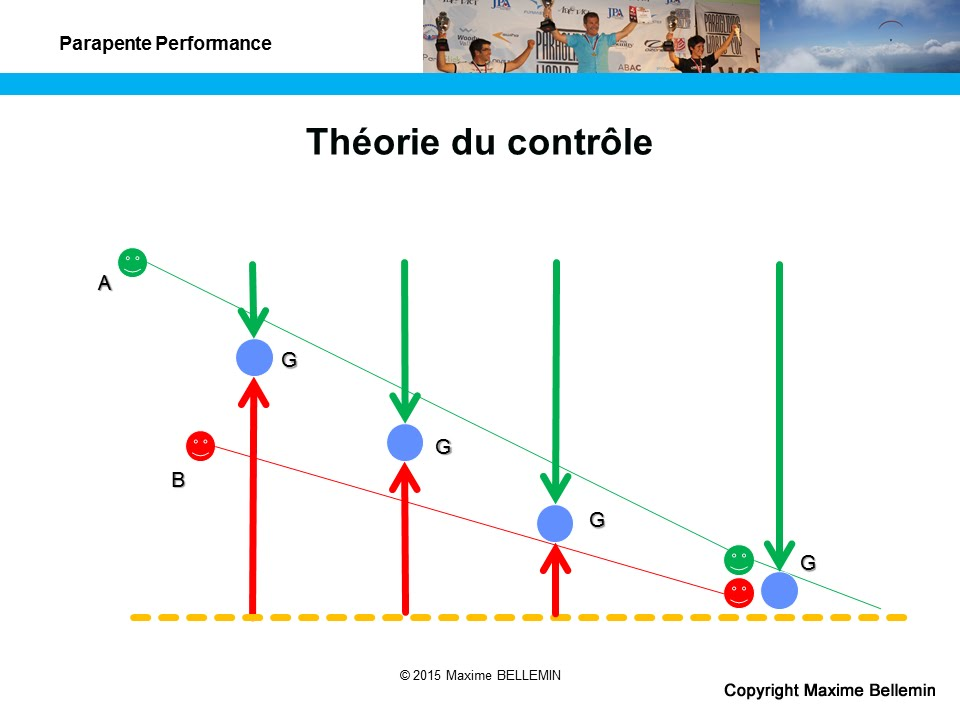# Theory of control: introduction

During a meeting with French paragliding coaches last year, Julien described the concept of control he develops with the pilots he manages at Pôle Espoir (a college facility mixing studies and sports practice). He introduced an equation supposed to define control. This formula teased me and seemed not to be reflecting all possible situations. On the other hand, the effort to present a mathematical solution to this problem revealed a strong and positive process that deserved being thoroughly studied.## Our situation

Let’s imagine two pilots flying. Pilot A is higher than pilot B. Pilot B is further on course than pilot A. Who controls who? What are the variables involved in determining that control? This series of blog entries provides theoretical elements to answer these questions.

If I am pilot A, depending on the altitude difference between me and pilot B, I’ll let him take a small lead on the course. Enough to let him search thermals for me but not too much to stay on top of him and with the ability to complete the task before him. By dividing the gap distance by the height difference, we get a glide ratio. The control glide ratio, which is often incorrectly called control angle.

Both A and B pilots seek to reach a position (not a spot) we will call G. We will take the assumption that, seen from very high, A and B pilots as well as G position are aligned. We will assume that B is closest to G while A is higher than B. All this is illustrated in the drawing above. Altitude of G position depends on parameters such as the respective initial positions of A and B, their flight behavior, the vario values of the day. Depending on the circumstances, G defines the boundary between the area controlled by A and the one controlled by B. Another strong assumption: our pilots fly in a neutral air mass (between thermals): no wind, no subsidence or restitution, no dynamic support, nada. Sometimes we will take the assumption that our pilots follow a rational behavior, but not always, it remains paragliding practice.

## Our assumptions

We claim that A controls B if he can get to G position or above G position before his fellow pilot:

• Very close to B going in the direction of G, A does not control much unless being really higher than B and that he finds not strong thermal.
• Further on course, if B finds no thermal, he will land. Above and after that landing position, A can arrive quiet and smooth to enjoy absolute control.
• In between those two points, that is initial B position and the final landing place, the of G position that allows A to control B will progressively get lower. This altitude depends on initial conditions such dD and dH as well as Vzmc, Va, Vb (if B is not the rational kind) and of course D.

Before generalizing the problem and its theory, let’s study its boundary conditions in two particular cases. In all equations I use equalities to determine the equilibrium point. In practice you have to understand them as « less than » when we consider the control glide ratio: for pilot A to control pilot B, A must see B with a glide ratio lower than that determined by the equilibrium equations.

## Our variables

Variables of our problem are the following:

• dH is the altitude difference in between A and B
• dD is the distance difference in between A and B
• H is the altitude that A will loose
• D is the distance that A will cover
• Va is the horizontal speed of A
• Vb is the horizontal speed of B
• Vmax is the maximum horizontal speed
• Vmc is the McCready horizontal speed
• Vza is the sink rate of A on course
• Vzb is the sink rate of B on course
• Vzmc is the expected climb rate
• Fa is the glide ratio on course of A
• Fb is the glide ratio on course of B
• Fmc is the glide ratio at McCready speed
• Fvmax is the glide ratio at maximum speed

All values are positive. Sink rate while in transition is positive, climb rate in thermal is positive. I kept symbols and diagrams in French. They should be legible. H could stand for height, V for velocity, D for distance, d for difference or delta. F comes out of Finesse which means glide ratio in French. You only need to get used to that important F letter.

## Our target

What we intend to determine is:

• Fc is the control glide ratio and equals to dD / dH

## 1 réflexion sur « Theory of control: introduction »

Les commentaires sont fermés.